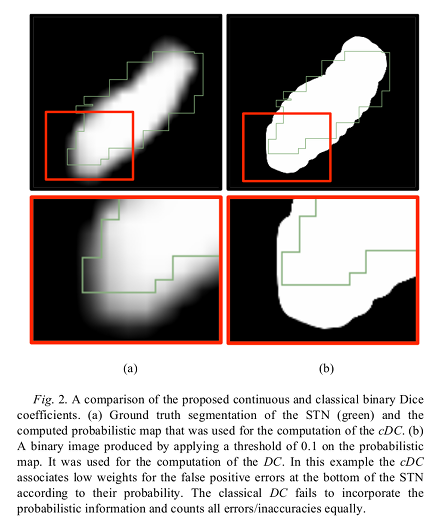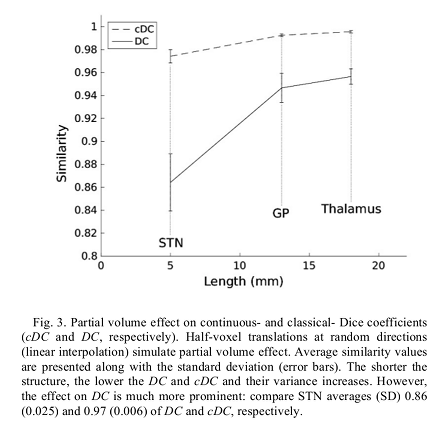# Highlights

Authors propose to use a variant of the Dice coefficient to evaluate continuous/probabilistic segmentations.

# Introduction

Overlapping measures are often utilized to quantify the similarity between two binary regions. However, modern segmentation algorithms output a probability or confidence map with continuous values in the zero-to-one interval. These maps have to be binarized/thresholded to use the Dice coefficient.

Also, it has been shown that the Dice coefficient is directly related to structure’s size: the smaller the structure the lower the Dice coefficient (given a fixed resolution). Therefore, Dice is a difficult measure for comparing methods tested on different structures.

Authors extend the definition of the classical Dice coefficient (DC) overlap to facilitate the direct comparison of a ground truth binary image with a probabilistic map. They call the extended method continuous Dice coefficient (cDC).

# Methods

Classical Dice coefficient $$DC := \frac{2 \lvert A \cap B \rvert}{\lvert A \rvert + \lvert B \rvert}$$

The classical DC fails to incorporate the probabilistic information and counts all errors/inaccuracies equally.

The proposed score cDC score: $$cDC := \frac{\lvert A \cap B \rvert}{c\lvert A \rvert + \lvert B \rvert}$$

where $$c$$ is defined as the mean value of B over the voxels where both A and B are positive: $$c = \frac{\sum_{i} a_{i} b_{i}}{\sum_{i} a_{i} sign(b_{i})}$$ and $$sign(x)$$ is defined as $$sign(x) = { \begin{cases} 1 &\; \text{if}\; x > 0\\ 0 &\; \text{if}\; x = 0\\ -1 &\; \text{if}\; x < 0 \end{cases} }$$

If $$\sum_{i} a_{i} sign(b_{i} = 0$$ (no overlap between A and B), authors arbitrary define $$c = 1$$. cDC will be zero in that case.

When $$b \in {0,1}$$, (i.e., a binary value) $$c = 1$$ and $$cDC = DC$$, and thus cDC is a consistent extension of DC.

Properties:

• $$cDC \leq 1$$ and $$cDC = 1$$ iff overlap is complete.
• cDC is monotonically decreasing with the amount of overlap.

## Data

Authors used gold-standard segmentations of the right subthalamic nucleus (STN), globus pallidus (GP), and thalamus on high-resolution 7T MRI brain images of a cohort of 46 Parkinsons’ disease patients to evaluate their method.

A threshold of 0.1 was applied to the segmentation probabilistic map to convert it into a binary image and compute the DC. This threshold was selected to achieve maximal DC value.

# Results# Conclusions

The cDC is well defined for probabilistic segmentation, less biased to the structure’s size and more robust to partial volume effects in comparison to DC.

Authors argue that as a better measurement tool, the method opens the door for the development of better segmentation methods.

# Remarks

It seems unclear how $$b_{i}$$ can be negative so that $$sign(x)$$ can take the value -1.

The described properties seem to be in contradiction.

A very limited set of experiments were performed.

The impact or benefit of the new score remains to be evaluated.